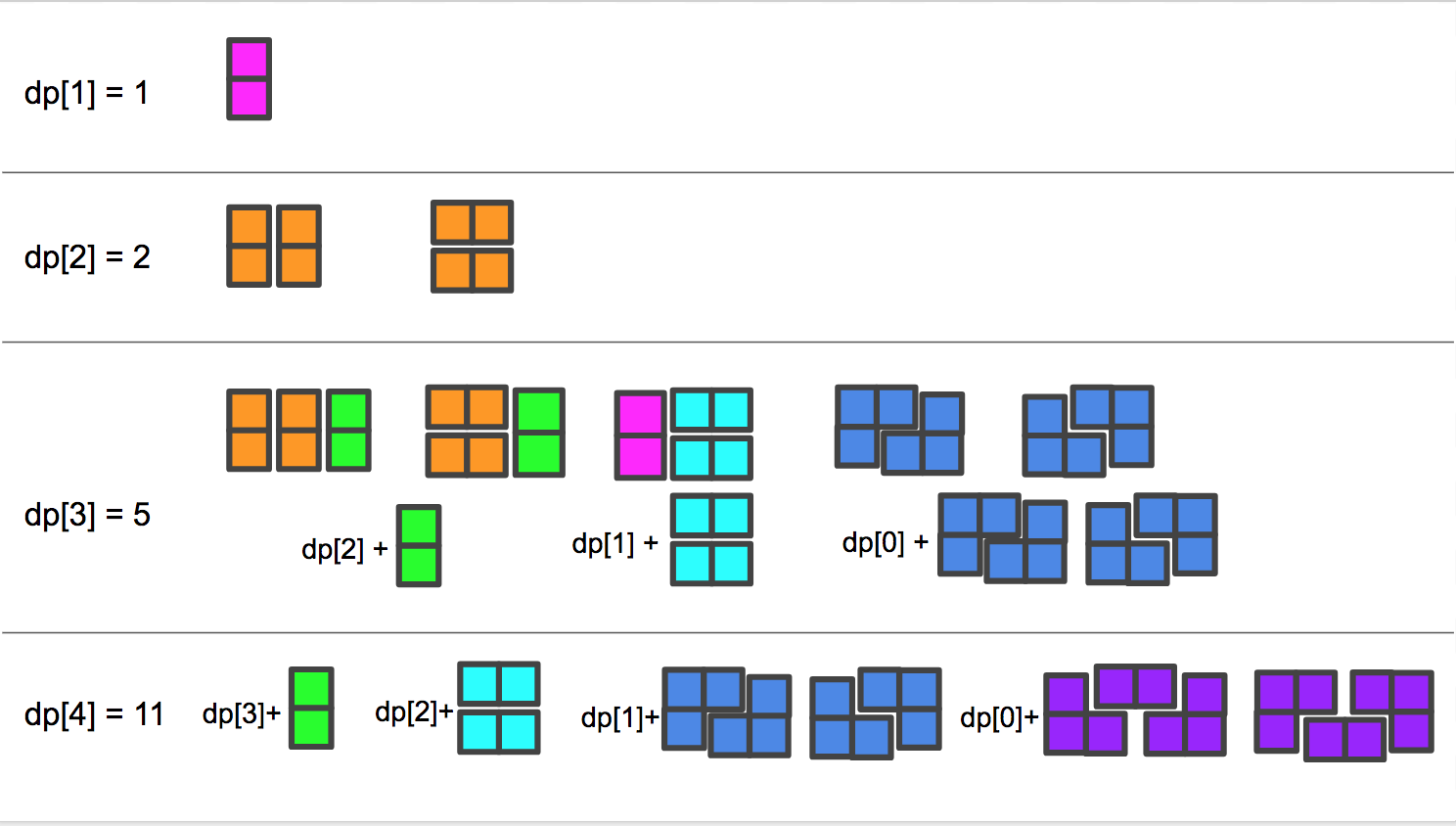# [LeetCode] Domino and Tromino Tiling 多米诺和三格骨牌

We have two types of tiles: a 2x1 domino shape, and an "L" tromino shape. These shapes may be rotated.

XX  <- domino

XX  <- "L" tromino
X


Given N, how many ways are there to tile a 2 x N board? Return your answer modulo 10^9 + 7.

(In a tiling, every square must be covered by a tile. Two tilings are different if and only if there are two 4-directionally adjacent cells on the board such that exactly one of the tilings has both squares occupied by a tile.)

Example:
Input: 3
Output: 5
Explanation:
The five different ways are listed below, different letters indicates different tiles:
XYZ XXZ XYY XXY XYY
XYZ YYZ XZZ XYY XXY

Note:

• N  will be in range [1, 1000].

N=5的情况博主没有再画了，可以参见ZhengKaiWei大神的帖子中的手稿图，很萌～

dp[n] = dp[n-1] + dp[n-2] + 2 * (dp[n-3] + ... + dp)

= dp[n-1] + dp[n-3] + dp[n-2] + dp[n-3] + 2 * (dp[n-4] + ... dp)

= dp[n-1] + dp[n-3] + dp[n-1]

= 2 * dp[n-1] + dp[n-3]class Solution {
public:
int numTilings(int N) {
int M = 1e9 + 7;
vector<long> dp(N + 1);
dp = 1; dp = 1; dp = 2;
for (int i = 3; i <= N; ++i) {
dp[i] = (dp[i - 1] * 2 + dp[i - 3]) % M;
}
return dp[N];
}
};

https://leetcode.com/problems/domino-and-tromino-tiling/discuss/116513/Java-solution-DP

https://leetcode.com/problems/domino-and-tromino-tiling/discuss/116581/Detail-and-explanation-of-O(n)-solution-why-dpn2*dn-1+dpn-3

https://leetcode.com/problems/domino-and-tromino-tiling/discuss/116664/Schematic-explanation-of-two-equivalent-DP-recurrence-formula

LeetCode All in One 题目讲解汇总(持续更新中...)

 微信打赏Venmo 打赏posted @ 2018-06-13 19:58  Grandyang  阅读(4183)  评论(1编辑  收藏  举报